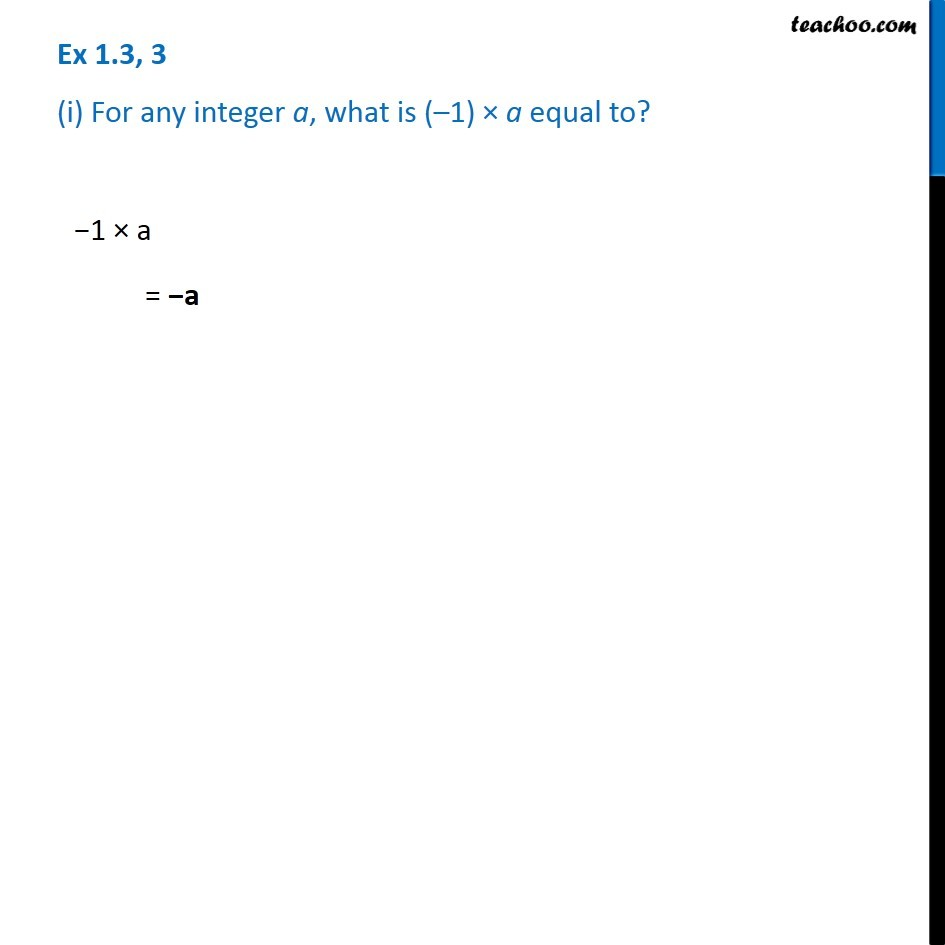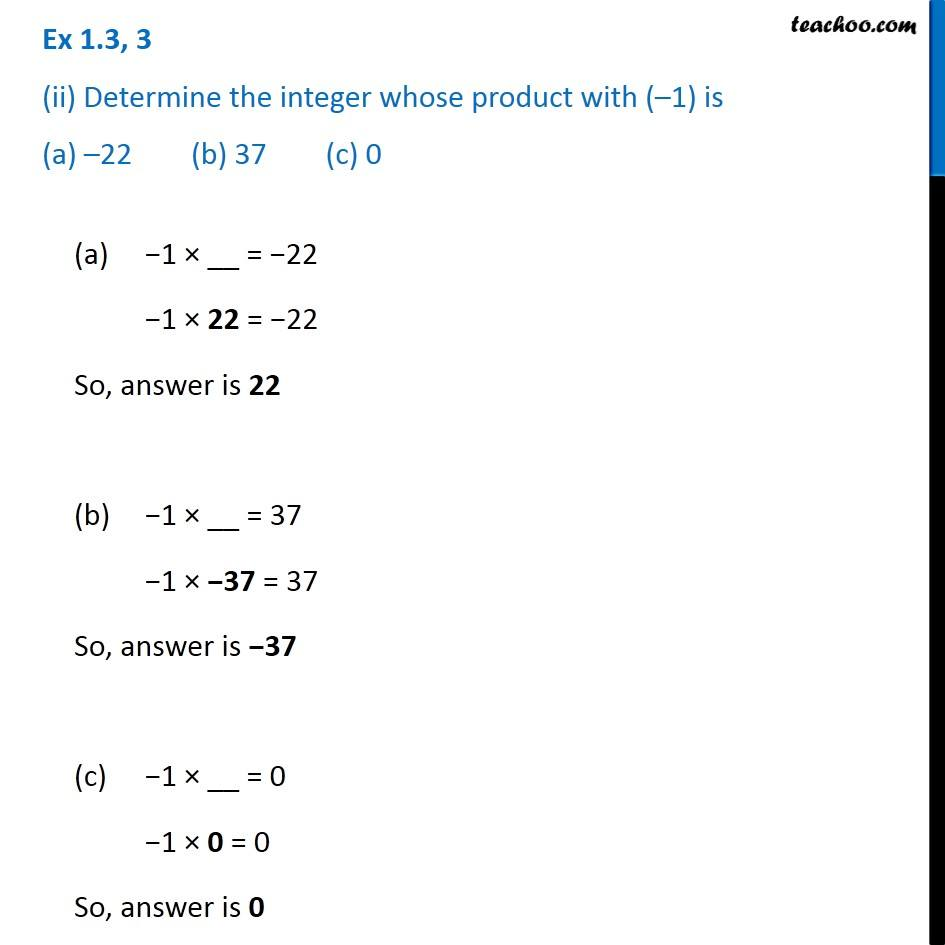Subscribe to our Youtube Channel - https://you.tube/teachoo

1. Chapter 1 Class 7 Integers
2. Serial order wise
3. Ex 1.3

Transcript

Ex 1.3, 3 (i) For any integer a, what is (–1) × a equal to? −1 × a = −a Ex 1.3, 3 (ii) Determine the integer whose product with (–1) is (a) –22 (b) 37 (c) 0 (a) −1 × __ = −22 −1 × 22 = −22 So, answer is 22 (b) −1 × __ = 37 −1 × −37 = 37 So, answer is −37 (c) −1 × __ = 0 −1 × 0 = 0 So, answer is 0

Ex 1.3

Chapter 1 Class 7 Integers
Serial order wise